PHY294H - Lecture 33

Steady state response - Driven LRC circuit

Consider an AC source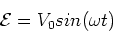(1)

applied to a series LRC combination. We shall consider the case in which this source has been applied to the circuit for long enough time for all transients to have dissappeared. In this limit, the current in the circuit has the same frequency as the AC source, however it may have a different phase, as was the case for the simple inductor and capacitor examples. Kirchhoff's law implies,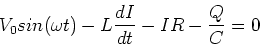(2)

or,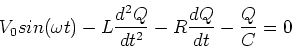(3)

We shall solve for the charge as a function of time and from it find the current using I = dQ/dt. The steady state solution to the equation above is,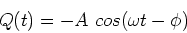(4)

In this problem, the constants A and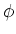are found by subsituting this assumption back into the equation above. Note that this is different than the transition LRC case where A andwere determined by the initial conditions. Here we are looking at the steady state of a driven system and this enables us to find A andin general. We shall find the current,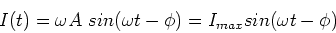(5)

Finding A andWe shall need two trigonometric identities,(6)

and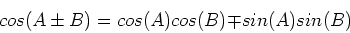(7)

We then write,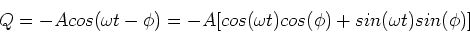(8)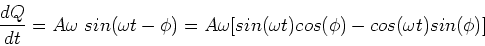(9)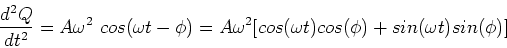(10)

We now use these expressions in Eq. (3) and set the coefficient of the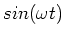term to zero, which gives,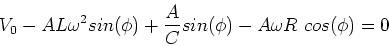(11)

Setting the coefficent of the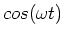term to zero yields,(12)

The latter equation yields,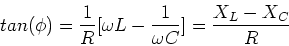(13)

This also implies that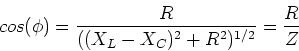(14)

where Z is the impedance, defined by,

 Z = (R2+(XL - XC)2)1/2. (15)

We also have,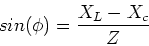(16)

Using these relations in Eq. (11) we have,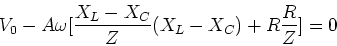(17)

Solving for A we find,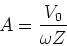(18)

The current in the circuit is then given by,(19)

The voltage across the resistor is given by,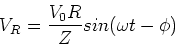(20)

The voltage across the capacitor is,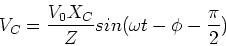(21)

The voltage across the inductor is,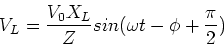(22)

Resonance

If we plot the phase angle and the impedance as a function of frequency, it is evident that there is a special frequency at which the impedance is small. This special frequency occurs when the capacitive and inductive reactances cancel,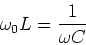(23)

This special frequency is just the natural frequency of the LC combination, ie.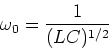(24)

At resonance, the phase angle is zero so the current is in phase with the source.